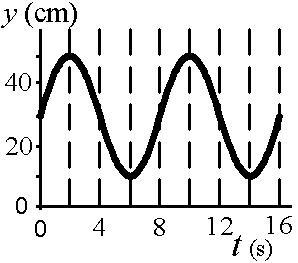# Problem: The diagram shows the motion of a simple harmonic oscillator.a. What is the amplitude of the motion (in cm)?b. What is the period (in s)?c. What is the equilibrium position (in cm)?d. What is the maximum velocity of the object (in cm/s)?

###### FREE Expert Solution

a.

Amplitude is the maximum or minimum displacement.

From the figure:

89% (121 ratings)###### Problem Details

The diagram shows the motion of a simple harmonic oscillator.a. What is the amplitude of the motion (in cm)?

b. What is the period (in s)?

c. What is the equilibrium position (in cm)?

d. What is the maximum velocity of the object (in cm/s)?